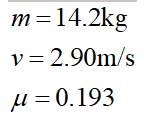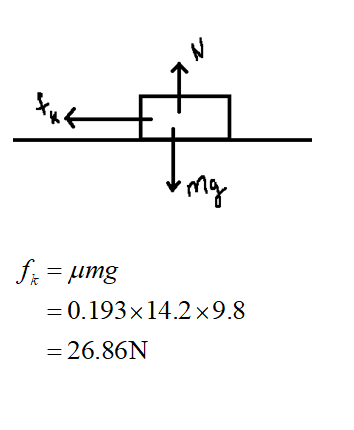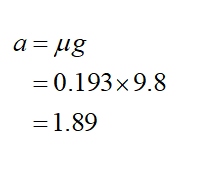# A block of mass 14.2 kg is sliding at an initial velocity of 2.90 m/s in the positive x-direction. The surface has a coefficient of kinetic friction of 0.193. (Indicate the direction with the signs of your answers.) (a)What is the force of kinetic friction (in N) acting on the block? N(b)What is the block's acceleration (in m/s2)? m/s2(c)How far will it slide (in m) before coming to rest? m

Question
148 views
A block of mass 14.2 kg is sliding at an initial velocity of 2.90 m/s in the positive x-direction. The surface has a coefficient of kinetic friction of 0.193. (Indicate the direction with the signs of your answers.)

(a)
What is the force of kinetic friction (in N) acting on the block?
N
(b)
What is the block's acceleration (in m/s2)?
m/s2
(c)
How far will it slide (in m) before coming to rest?
m
check_circle

Step 1

It is given thatStep 2
1. a) The force of kinetic friction acting on the block is given as
2.Step 3

b)

Acceleration is given as...

### Want to see the full answer?

See Solution

#### Want to see this answer and more?

Solutions are written by subject experts who are available 24/7. Questions are typically answered within 1 hour.*

See Solution
*Response times may vary by subject and question.
Tagged in